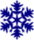# Obeleži sve kategorije koje odgovaraju problemu

### Još detalja - opišite nam problem

Uspešno ste prijavili problem!
Status problema i sve dodatne informacije možete pratiti klikom na link.
Nažalost nismo trenutno u mogućnosti da obradimo vaš zahtev.
Molimo vas da pokušate kasnije.

# Moving multiple objects¶

So far, we have made animations in which various objects were moving (car, billiard ball, plane, text), but in each of these programs there was only one moving object. For the global variables that describe the scene we used the coordinates of that moving object, and sometimes we also used other values, such as displacement, direction of motion, and so on.

The movement of multiple objects is not fundamentally different from the movement of a single object - we will simply need to track values that describe movement for all of the objects. For example, we can represent each object with a tuple of values describing it, and keep all such tuples in a list.

## Balls¶

In the following example we will see animated motion of several balls. Each ball is represented by a $$(x, y, dx, dy, r, color)$$ tuple, where $$x, y$$ are the coordinates of the center of the ball, $$dx, dy$$ are the displacements of the ball per coordinate, $$r$$ is the radius, and $$color$$ is the color of the ball. All such tuples are placed in the list balls.

When unpacking a tuple from a list element (command x, y, dx, dy, r, color = balls[i]), as well as when returning it to the list (command balls[i] = (x, y, dx, dy, r, color)), we should mind the order of the variables.

In the example, we use the random module to create the balls in order to get random selections (imported using the import command). The random.randint(a, b) function returns a random integer between a and b (including boundaries), and the random.choice(a) function returns a random element from the collection a.

This example is given primarily to introduce a different way of working with a list of tuples that describe a scene. Because of that, some interesting details were left in the background. Let us look a bit at those, in this context, side details. To get the effect of approaching, the stars in front of us should move apart and magnify. Therefore, in the program, the values of x and y change so that the stars move from the center of the window to the edges, moving faster as they go further away from the center.

It’s not particularly important that you fully understand the commands that change the values of x, y, and r in order to learn programming, but you can try out how the animation changes when you slightly alter the coefficients in those commands (for example, stars can be moving slower, or growing faster).

####Snowflakes: Complete the program so that it works as shown in the example (“Play Task” button).

Each snowflake is described with only two numbers, its coordinates, so the tuples we will use will actually be pairs $$(x, y)$$.

Snowflakes fall 1 pixel at a time, and those that go out of the window are replaced with new snowflakes. Forming a new state is similar to that of the “stars” program, only the rules for moving snowflakes are simpler.

The snowflakes in the initial set are selected anywhere in the window, and the ones that are added later are selected somewhere on the top edge of the window.####Gliding text Try out the program and try to understand how it works. Try altering something in the program (the text being displayed, the color in which the text is being displayed, the speed at which the text moves, or any other detail).

Challenge: Try to modify the program so that the text glides down instead of up.